# Trigonometric Table

Trigonometric Table
Go back to  'Trigonometry'

Trigonometry deals with the measurement of angles and problems related to angles.

The term trigonometry is derived from a Latin word.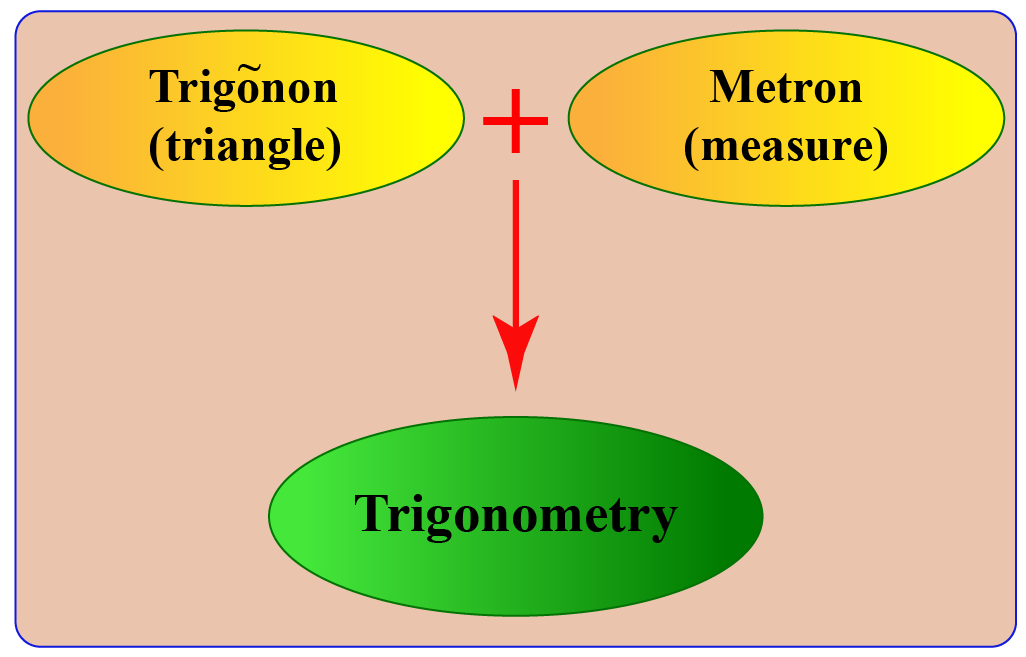Trigonometry makes use of the relationship between the angles and sides of a triangle.

As you scroll down, you'll learn about trigonometric values, standard angles, trigonometric tables, etc.

## Lesson Plan

 1 What Are the Trigonometric Values? 2 Challenging Questions on Trigonometric Table 3 Important Notes on Trigonometric Table 4 Solved Examples on Trigonometric Table 5 Interactive Questions on Trigonometric Table

## Unit Circle and Trigonometric Values

Explore the trigonometric values using this interactive unit circle

Move the point around to see the values changing and their relationship with the length of the sides of the triangle.

Trigonometry deals with the relationship between the sides of a triangle (right-angled triangle) and its angles.

The trigonometric values is a collective term for values of different ratios, such as sine, cosine, tangent, secant, cotangent, and cosecant.

All the trigonometric functions are related to the sides of a right-angle triangle and their values are found using the following ratios.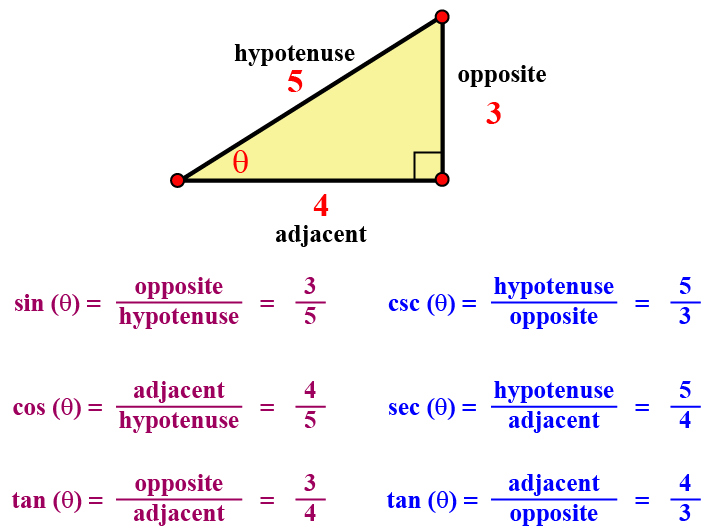To remember this easily remember the word "SOHCAHTOA"!

• SOH

Sine = Opposite / Hypotenuse

• CAH

• TOA

## Standard Angles

The trigonometric values for the angles 0°, 30°, 45°, 60°, and 90° are commonly used to solve the trigonometry problems.

These values are associated with the measurement of the lengths and the angles of a right-angle triangle.

Thus, 0°, 30°, 45°, 60°, and 90° are called the standard angles in trigonometry.

## Trigonometric Table

Values of trigonometric functions for the standard angles such as 0°, 30°, 45°, 60°, and 90° could be easily found using a trigonometric ratios table.

The table consists of trigonometric ratios – sine, cosine, tangent, cosecant, secant, and cotangent.

In short, these ratios are written as sin, cos, tan, cosec, sec, and cot.

It is best to remember the values of the trigonometric ratios of these standard angles.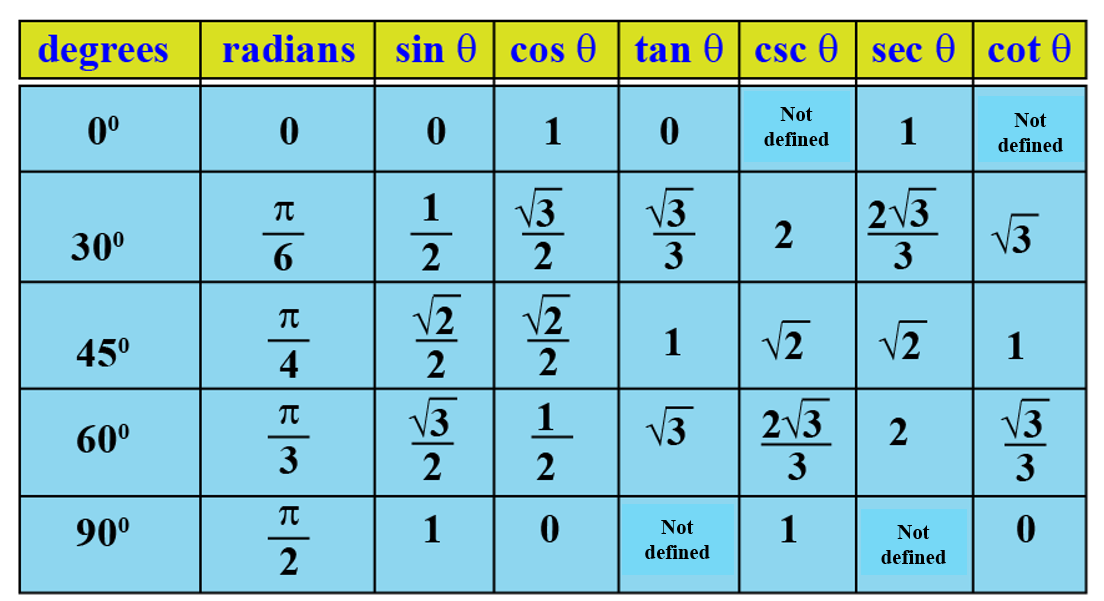Using the above table, values of sin pi/6, cos pi/6, tan pi/6, sin pi/4, cos pi/4, tan pi/4, sin pi/3, cos pi/3, tan pi/3, sin pi/2, cos pi/2, and tan pi/2 in radians could be easily found out.

A trigonometric table has wide application in fields like science and engineering.

### Trick to Remember Trigonometric Table

Let us learn the one-hand trick for remembering the trigonometric table easily!

Designate each finger the standard angles as shown in the image. For filling the sine table we will include counting of the fingers, while for the cos table we will simply fill the values in reverse order.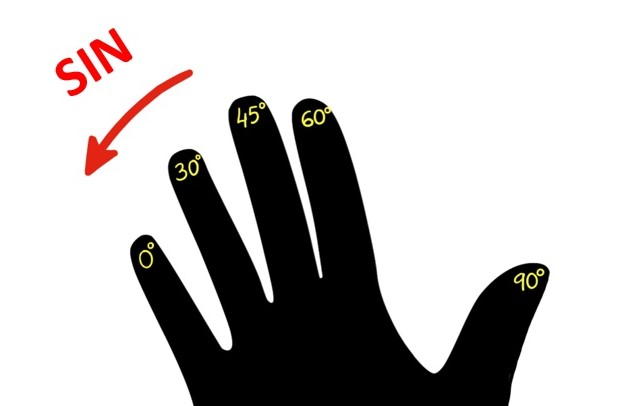Step 1: For the sine table, count the fingers on the left side for the standard angle.

Step 2: Divide the number of fingers by 4

Step 3: Take out the square root of the ratio.

Example 1: For sin 0°, there are no fingers on the left-hand side, therefore we will take 0. Dividing the zero by 4 we get 0. Taking the square root of the ratio we would get the value of sin 0° = 0

Example 2: For sin 60°, there are 3 fingers on the left-hand side. Dividing 3 by 4 we get $$\dfrac{3}{4}$$. Taking the square root of the ratio $$\sqrt {\dfrac34}$$ we would get the value of sin 30° = $$\dfrac {\sqrt 3}2$$

Similarly, we can find out values for sin 30°, 45°, and 90° to fill the table.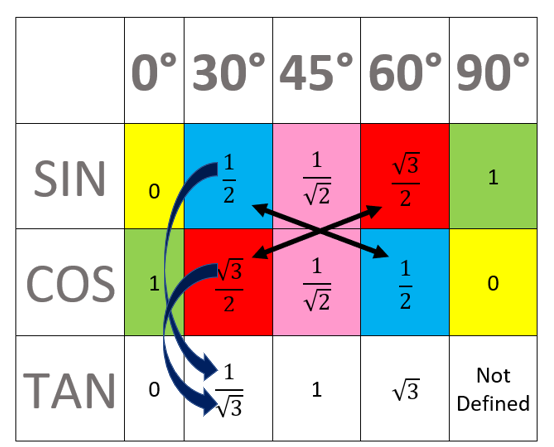In order to complete the cos table, simply fill in the values of sine in a reverse manner.

For tan values, create the ratios by taking numerators of sine and cos values of the corresponding angle as indicated by the arrows in the above picture.Challenging Questions
1. What will be the trigonometric values of negative angles?
2. A right-angled triangle has an area of 400 square units. What will be the length of its hypotenuse

## Understanding the Trigonometric Table

Let us consider a unit circle to understand the concept of trigonometry ratios.

The center of the circle is located at the origin (0,0).

The radius of the circle is equal to 1.

We can directly measure the angles of cos, sin, and tan with the help of the unit circle.

Let the angle be $$\theta$$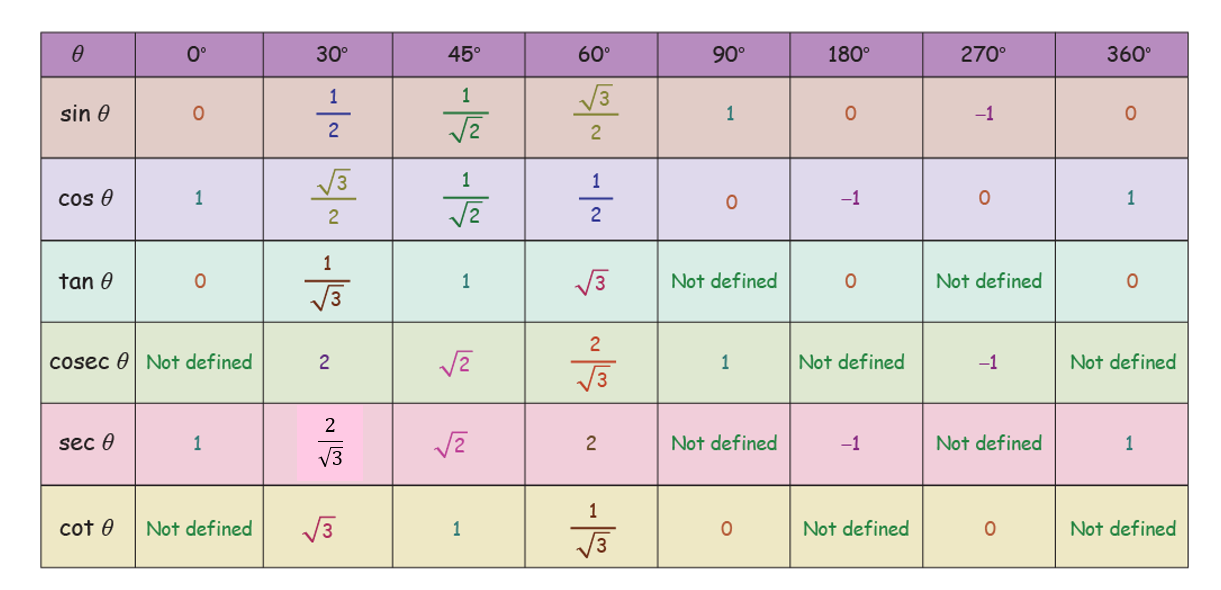Important Notes
1. Trigonometric values are based on the three major trigonometric ratios: Sine, Cosine, and Tangent.

Sine or sin $$\theta$$ = Side opposite to $$\theta$$ / Hypotenuse
Cosines or cos $$\theta$$ = Adjacent side to $$\theta$$ / Hypotenuse
Tangent or tan $$\theta$$ =Side opposite to $$\theta$$ / Adjacent side to $$\theta$$

2. 0°, 30°, 45°, 60°, and 90° are called the standard angles in trigonometry.

## Solved Examples

 Example 1

Can you help Jonathan to find out the exact value of $$\sin15^{\circ}$$?

Solution

\begin{align} \sin15^{\circ} &=\sin45^{\circ}-30^{\circ})\\ &=\sin45^{\circ} \cos30^{\circ} - \cos45^{\circ} \sin30^{\circ}\\ &= \dfrac{\sqrt2}2 . \dfrac{\sqrt3}2 + \dfrac{\sqrt2}2 . \dfrac 12 \\ &= \dfrac {\sqrt6 + \sqrt 2}{4}\end{align}

 $$\therefore$$ The value of $$\sin15^{\circ} = \dfrac {\sqrt6 + \sqrt 2}{4}$$
 Example 2

Esther challenges her students to find out the height of the glacier. Students are allowed to use the calculator. Can you determine it?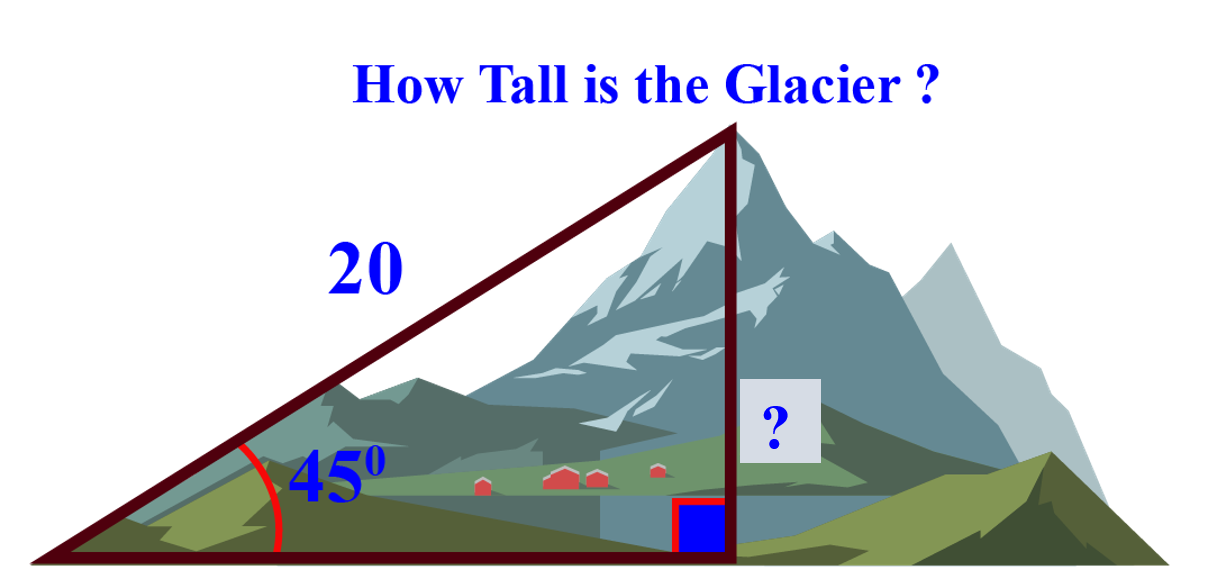Solution

The length of the hypotenuse is known to be 20 units.

We want to find out the opposite side to the angle.

Sine ratio uses the opposite side and hypotenuse.

Remember the word "SOHCAHTOA"!

SOH

Sine = Opposite / Hypotenuse

\begin{align}\sin 45^{\circ} &= \dfrac {\text {Opposite}}{\text {Hypotenuse}}\\ \dfrac {1}{\sqrt2} &= \dfrac {\text {Opposite}}{20}\\ \text {Opposite} &= \dfrac {20}{\sqrt2}\\ \text {Opposite} &\approx 14.14\end{align}

 $$\therefore$$ The height of the glacier is approx. 14.14 units.
 Example 3

Help Ron to write the values of:

(a) \begin{align}\sin \frac{\pi }{6}\end{align}

(b) \begin{align}\sec \frac{\pi }{3}\end{align}

(c) \begin{align}\cot \frac{\pi }{2}\end{align}

Solution

The trigonometric ratios table helps us to find the above values quickly.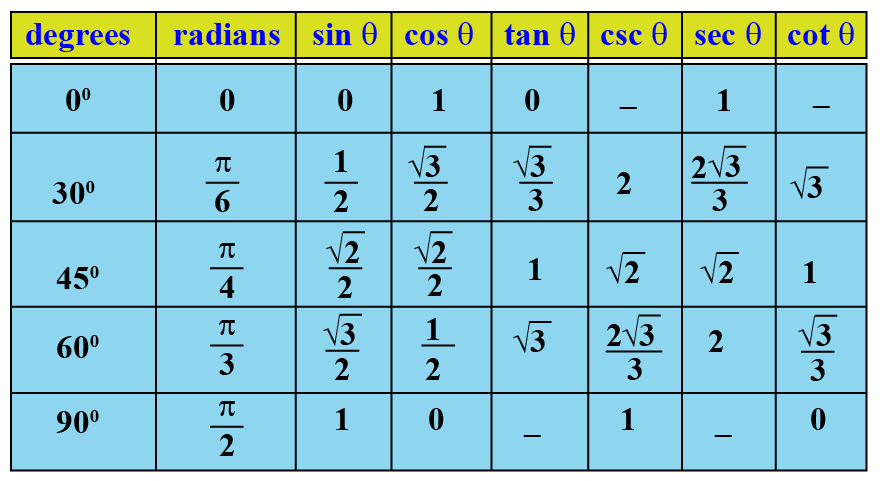We have:

(a) \begin{align}\sin \frac{\pi }{6} = \sin {30^0} = \frac{1}{2}\end{align}

(b) \begin{align}\sec \frac{\pi }{3} = \sec {60^0} = 2\end{align}

(c) \begin{align}\cot \frac{\pi }{2} = \cot {90^0} = 0\end{align}

 (a) $$\dfrac{1}{2}$$   (b) $$2$$   (c) $$0$$

## Interactive Questions

Here are a few activities for you to practice. Select/Type your answer and click the "Check Answer" button to see the result.

## Let's Summarize

The mini-lesson targeted the fascinating concept of a trigonometric table. The math journey around the trigonometric table starts with what a student already knows, and goes on to creatively crafting a fresh concept in the young minds. Done in a way that not only it is relatable and easy to grasp, but also will stay with them forever. Here lies the magic with Cuemath.

At Cuemath, our team of math experts is dedicated to making learning fun for our favorite readers, the students!

Through an interactive and engaging learning-teaching-learning approach, the teachers explore all angles of a topic.

Be it worksheets, online classes, doubt sessions, or any other form of relation, it’s the logical thinking and smart learning approach that we, at Cuemath, believe in.

## 1. What is the sin cos table?

Trigonometric ratios table or sin cos table gives the values of trigonometric functions for the standard angles such as 0°, 30°, 45°, 60°, and 90° in a tabulated manner.

## 2. What are the standard angles?

Trigonometric values for the angles 0°, 30°, 45°, 60°, and 90° are commonly used to solve the trigonometry problems.

These values are associated with the measurement of lengths and angles of the right-angle triangle.

Thus, 0°, 30°, 45°, 60°, and 90° are called the standard angles in trigonometry.

## 3. What does the infinity value of the trigonometric ratio mean?

Undefined values are often given an infinity value because there is no definite value to assign them at this point.

More Important Topics
Numbers
Algebra
Geometry
Measurement
Money
Data
Trigonometry
Calculus